# Graph Info

The GripQL API allows a user to download the schema of a graph. This outlines the different types of nodes, the edges the connect them and the structure of the documents stored in graph elements. A graph document has a `graph` field that has the name, a `vertices` field and an `edges` field.

``````{
"graph": "rc5",
"vertices": [
{"gid": "Compound",
"label": "Compound",
"data": {"name": "STRING", "term": "STRING", "term_id": "STRING"}},
...],
"edges": [
{"gid": "(Project)--program->(Program)",
"label": "program",
"from": "Project",
"to": "Program",
"data": {}}
...]
}
``````

Connect to BMEG server

``````import networkx as nx
import matplotlib.pyplot as plt
import gripql
from networkx.drawing.nx_agraph import graphviz_layout
``````
``````conn = gripql.Connection("https://bmeg.io/api", credential_file="bmeg_credentials.json")
``````

Print avalible graphs

``````print(conn.listGraphs())
``````
``````['bmeg_rc1_3', 'bmeg_rc1_3__schema__', 'bmeg_rc2', 'bmeg_rc3', 'bmeg_rc2__schema__', 'rc4', 'bmeg_rc3__schema__', 'rc4__schema__', 'rc5', 'rc5__schema__']
``````

Get the schema graph

``````schema = conn.getSchema("rc5")
``````

Start build graph using NetworkX

``````g = nx.MultiDiGraph()
``````
``````for v in schema['vertices']:
for e in schema['edges']:
``````

Draw Schema Graph

``````pos = graphviz_layout(g, prog='twopi', args='')
fig, ax = plt.subplots(1, 1, figsize=(8, 6));
nx.draw(g, pos, ax=ax, with_labels=True)
``````
``````/usr/local/lib/python3.7/site-packages/networkx/drawing/nx_pylab.py:563: MatplotlibDeprecationWarning:
The iterable function was deprecated in Matplotlib 3.1 and will be removed in 3.3. Use np.iterable instead.
if not cb.iterable(width):
/usr/local/lib/python3.7/site-packages/networkx/drawing/nx_pylab.py:660: MatplotlibDeprecationWarning:
The iterable function was deprecated in Matplotlib 3.1 and will be removed in 3.3. Use np.iterable instead.
if cb.iterable(node_size):  # many node sizes
``````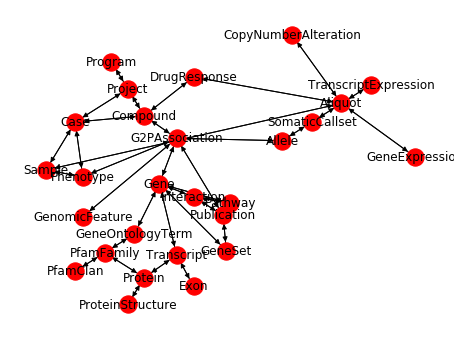``````
``````

# Allele Lookup

``````import hashlib
import gripql
conn = gripql.Connection("https://bmeg.io/api", credential_file="bmeg_credentials.json")
G = conn.graph("rc5")
``````

BMEG stores alleles using a hashed version of the alteration description

``````genome:chromosome:start:reference_bases:alternate_bases
``````

So an example allele would be:

``````GRCh37:1:27100988:C:T
``````

Which is then run though a `sha1` hash to get the string

``````0b0a7a23d57414e768677a6cbd764563922209df
``````
``````def allele_gid(genome: str, chromosome: str, start: int,
reference_bases: str, alternate_bases: str):

if not all(v is not None for v in [genome, chromosome, start,
reference_bases, alternate_bases]):
raise ValueError("one or more args was None")

start = int(start)
if reference_bases == "-" or alternate_bases == "-":
pass
elif reference_bases != alternate_bases:
pass
elif len(reference_bases) > len(alternate_bases):
common = os.path.commonprefix([reference_bases, alternate_bases])
reference_bases = reference_bases[len(common):]
if len(alternate_bases) == len(common):
alternate_bases = "-"
else:
alternate_bases = alternate_bases[len(common):]
start += len(common)
elif len(reference_bases) < len(alternate_bases):
common = os.path.commonprefix([reference_bases, alternate_bases])
alternate_bases = alternate_bases[len(common):]
if len(reference_bases) == len(common):
reference_bases = "-"
else:
reference_bases = reference_bases[len(common):]

vid = "{}:{}:{}:{}:{}".format(genome, chromosome, start, reference_bases, alternate_bases)
vid = vid.encode('utf-8')
vidhash = hashlib.sha1()
vidhash.update(vid)
vidhash = vidhash.hexdigest()
return "Allele:{}".format(vidhash)
``````
``````chrom = 1
loc = 27100988
ids = []
for r in ['A', 'C', 'G', 'T']:
for a in ['A', 'C', 'G', 'T']:
ids.append( allele_gid("GRCh37", chrom, loc, r, a) )
for row in G.query().V(ids):
print( row )
``````
``````[INFO]  2020-01-14 13:28:17,360 1 results received in 0 seconds

<AttrDict({'gid': 'Allele:a457a505c1551c4dbd936cddb388b021422bc0bc', 'label': 'Allele', 'data': {'all_effects': 'ARID1A,stop_gained,p.Gln1424Ter,ENST00000324856,NM_006015.4;ARID1A,stop_gained,p.Gln1041Ter,ENST00000374152,;ARID1A,stop_gained,p.Gln321Ter,ENST00000430799,;ARID1A,intron_variant,,ENST00000457599,NM_139135.2;ARID1A,intron_variant,,ENST00000540690,;ARID1A,intron_variant,,ENST00000466382,;ARID1A,upstream_gene_variant,,ENST00000532781,;', 'alternate_bases': 'T', 'amino_acids': 'Q/*', 'biotype': 'protein_coding', 'cdna_position': '4641/8577', 'cds_position': '4270/6858', 'chromosome': '1', 'codons': 'Cag/Tag', 'end': 27100988, 'ensembl_gene': 'ENSG00000117713', 'ensembl_protein': 'ENSP00000320485', 'ensembl_transcript': 'ENST00000324856', 'exon_number': '18/20', 'genome': 'GRCh37', 'hgnc_id': '11110', 'hgvsc': 'c.4270C>T', 'hgvsp': 'p.Gln1424Ter', 'hgvsp_short': 'p.Q1424*', 'hugo_symbol': 'ARID1A', 'impact': 'HIGH', 'project_id': 'Project:Reference', 'protein_position': '1424/2285', 'reference_bases': 'C', 'start': 27100988, 'strand': '+', 'submitter_id': None, 'variant_classification': 'Nonsense_Mutation', 'variant_type': 'SNP'}})>
``````

# Gene Info

``````import gripql
conn = gripql.Connection("https://bmeg.io/api", credential_file="bmeg_credentials.json")
G = conn.graph("rc5")
``````

Look up a gene by its hugo symbol

``````gids = G.query().V().hasLabel("Gene").has(gripql.eq("\$.symbol", "BRCA1")).render("_gid").execute()
``````
``````[INFO]  2020-01-14 14:11:07,060 1 results received in 0 seconds
``````

Find some of the Gene Ontology terms the gene is linked to

``````for ent in G.query().V(gids).out("gene_ontology_terms").limit(10):
print(ent.gid, ent.data.definition)
``````
``````[INFO]  2020-01-14 14:11:09,590 10 results received in 0 seconds

GeneOntologyTerm:GO:0000724 The error-free repair of a double-strand break in DNA in which the broken DNA molecule is repaired using homologous sequences. A strand in the broken DNA searches for a homologous region in an intact chromosome to serve as the template for DNA synthesis. The restoration of two intact DNA molecules results in the exchange, reciprocal or nonreciprocal, of genetic material between the intact DNA molecule and the broken DNA molecule.
GeneOntologyTerm:GO:0000800 A proteinaceous core found between sister chromatids during meiotic prophase.
GeneOntologyTerm:GO:0003713 A protein or a member of a complex that interacts specifically and non-covalently with a DNA-bound DNA-binding transcription factor to activate the transcription of specific genes. Coactivators often act by altering chromatin structure and modifications. For example, one class of transcription coregulators modifies chromatin structure through covalent modification of histones. A second ATP-dependent class modifies the conformation of chromatin. Another type of coregulator activity is the bridging of a DNA-binding transcription factor to the basal transcription machinery. The Mediator complex, which bridges transcription factors and RNA polymerase, is also a transcription coactivator.
GeneOntologyTerm:GO:0003723 Interacting selectively and non-covalently with an RNA molecule or a portion thereof.
GeneOntologyTerm:GO:0004842 Catalysis of the transfer of ubiquitin from one protein to another via the reaction X-Ub + Y --> Y-Ub + X, where both X-Ub and Y-Ub are covalent linkages.
GeneOntologyTerm:GO:0005515 Interacting selectively and non-covalently with any protein or protein complex (a complex of two or more proteins that may include other nonprotein molecules).
GeneOntologyTerm:GO:0005634 A membrane-bounded organelle of eukaryotic cells in which chromosomes are housed and replicated. In most cells, the nucleus contains all of the cell's chromosomes except the organellar chromosomes, and is the site of RNA synthesis and processing. In some species, or in specialized cell types, RNA metabolism or DNA replication may be absent.
GeneOntologyTerm:GO:0005654 That part of the nuclear content other than the chromosomes or the nucleolus.
GeneOntologyTerm:GO:0005737 All of the contents of a cell excluding the plasma membrane and nucleus, but including other subcellular structures.
GeneOntologyTerm:GO:0006302 The repair of double-strand breaks in DNA via homologous and nonhomologous mechanisms to reform a continuous DNA helix.
``````
``````
``````

# Sample Counts

``````import gripql
conn = gripql.Connection("https://bmeg.io/api", credential_file="bmeg_credentials.json")
G = conn.graph("rc5")
``````

Count number of Projects per Program

``````q = G.query().V().hasLabel("Program").as_("p").out("projects").select("p")
q = q.aggregate(gripql.term("project_count", "\$._gid"))
for row in q.execute()["project_count"]["buckets"]:
print("%s\t%s" % (row["key"], row["value"]))
``````
``````[INFO]  2020-01-14 14:25:03,363 1 results received in 0 seconds

Program:TCGA    33
Program:GTEx    31
Program:CCLE    1
Program:GDSC    1
Program:CTRP    1
``````

Count number of Cases per Program

``````q = G.query().V().hasLabel("Program").as_("p").out("projects").out("cases").select("p")
q = q.aggregate(gripql.term("sample_count", "\$._gid"))
for row in q.execute()["sample_count"]["buckets"]:
print("%s\t%s" % (row["key"], row["value"]))
``````
``````[INFO]  2020-01-14 14:25:23,826 1 results received in 1 seconds

Program:TCGA    11315
Program:GTEx    8859
Program:CCLE    1618
Program:GDSC    1075
Program:CTRP    887
``````

Count number of Samples per Program

``````q = G.query().V().hasLabel("Program").as_("p").out("projects").out("cases").out("samples").select("p")
q = q.aggregate(gripql.term("sample_count", "\$._gid"))
for row in q.execute()["sample_count"]["buckets"]:
print("%s\t%s" % (row["key"], row["value"]))
``````
``````[INFO]  2020-01-14 14:26:37,527 1 results received in 13 seconds

Program:GTEx    204512
Program:TCGA    33405
Program:CCLE    1618
Program:GDSC    1075
Program:CTRP    887
``````
``````
``````

# Cohort Mutation Counts

Find the number of mutations per gene for TCGA cohort

``````import pandas
import gripql
conn = gripql.Connection("https://bmeg.io/api", credential_file="bmeg_credentials.json")
G = conn.graph("rc5")
``````

Select all the tumor samples in the TCGA KIRC cohort, and aggregate across the `ensembl_gene` field.

``````q = G.query().V("Project:TCGA-KIRC")
q = q.out("cases").out("samples").has(gripql.eq("gdc_attributes.sample_type", "Primary Tumor"))
q = q.out("aliquots").out("somatic_callsets").outE("alleles")
q = q.has(gripql.contains("methods", "MUTECT"))
q = q.aggregate(gripql.term("geneCount", "ensembl_gene"))

res = list(q)
counts = {}
for i in res['geneCount']['buckets']:
counts[i['key']] = i['value']

``````
``````[INFO]  2020-01-14 14:20:35,273 1 results received in 1 seconds
``````

Create a Pandas.Series with the output and find all the genes with 20 or more mutations

``````countDF = pandas.Series(counts)
``````
``````goi = list(countDF.index[countDF >= 20])
``````
``````for e,g in G.query().V(goi).render(["\$._gid" ,"\$.symbol"]):
print("%s (%s) = %d" % (e,g, countDF[e]))
``````
``````[INFO]  2020-01-14 14:20:42,933 10 results received in 0 seconds

ENSG00000007174 (DNAH9) = 22
ENSG00000081479 (LRP2) = 22
ENSG00000134086 (VHL) = 113
ENSG00000151914 (DST) = 27
ENSG00000155657 (TTN) = 120
ENSG00000163930 (BAP1) = 30
ENSG00000163939 (PBRM1) = 74
ENSG00000181143 (MUC16) = 42
ENSG00000181555 (SETD2) = 34
ENSG00000198793 (MTOR) = 34
``````
``````
``````

# Gene Mutation Hotstops

For this example, we will start from a single gene, and identify all mutations that occur on it.

``````import matplotlib.pyplot as plt
import pandas
import gripql
conn = gripql.Connection("https://bmeg.io/api", credential_file="bmeg_credentials.json")
G = conn.graph("rc5")
``````

Get BRCA1 start and stop locations

``````loc = G.query().V().hasLabel("Gene").has(gripql.eq("symbol", "BRCA1")).render(["\$.start", "\$.end"]).execute()
``````
``````[INFO]  2020-01-14 14:12:16,554 1 results received in 0 seconds
``````

Run an aggregation query to count up all the mutations

``````counts =  * (loc-loc)
q = G.query().V().hasLabel("Gene").has(gripql.eq("symbol", "BRCA1"))
q = q.out("alleles").has(gripql.and_(gripql.eq("variant_type", "SNP"),
gripql.gte("start", loc),
gripql.lte("end", loc)))
q = q.aggregate(gripql.term("brac1_pos", "start"))
res = list(q)
for v in res.brac1_pos.buckets:
counts[ v['key'] - loc ] = v['value']
``````
``````[INFO]  2020-01-14 14:17:12,740 1 results received in 0 seconds
``````

Save as a dataframe

``````s = pandas.DataFrame(counts)
``````

Plot the hotspots

``````s.rolling(500).sum().plot()
``````
``````<matplotlib.axes._subplots.AxesSubplot at 0x122303e10>
``````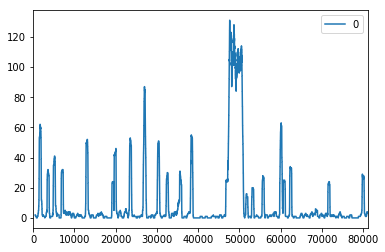``````
``````

# Cohort Genomics

Connect to BMEG server

``````import matplotlib.pyplot as plt
import gripql
conn = gripql.Connection("https://bmeg.io/api", credential_file="bmeg_credentials.json")
G = conn.graph("rc5")
``````

Do a query that starts on the TCGA BRCA cohort, goes though Cases -> Samples -> Aliquots -> SomaticCallsets -> Alleles. Once at the alleles, do an aggrigation to count the number of times each chromsome occurs

``````q = G.query().V("Project:TCGA-BRCA").out("cases").out("samples")
q = q.has(gripql.eq("gdc_attributes.sample_type", "Primary Tumor"))
q = q.out("aliquots").out("somatic_callsets").out("alleles")
q = q.has(gripql.eq("variant_type", "SNP"))
q = q.aggregate(gripql.term("chrom", "chromosome"))
res = q.execute()
``````
``````[INFO]  2020-01-14 14:24:22,453 1 results received in 9 seconds
``````

Visualize the results

``````name = []
count = []
for i in res.chrom.buckets:
name.append(i["key"])
count.append(i["value"])
plt.bar(name, count, width=0.35)
``````
``````<BarContainer object of 24 artists>
``````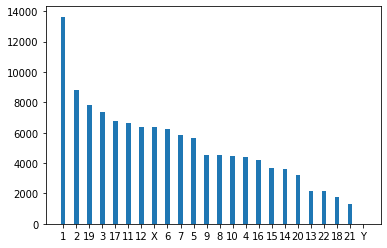``````
``````

# Expression Data

``````import seaborn as sns
import pandas
import gripql
conn = gripql.Connection("https://bmeg.io/api", credential_file="bmeg_credentials.json")
G = conn.graph("rc5")
``````

``````c = G.query().V("Project:TCGA-READ").out("cases").out("samples").as_("sample")
c = c.out("aliquots").out("gene_expressions").as_("exp")
c = c.render( ["\$sample._data.gdc_attributes.submitter_id", "\$exp._data.values"])

data = {}
for row in c.execute(stream=True):
data[row] = row
``````
``````[INFO]  2020-01-14 14:06:01,252 177 results received in 35 seconds
``````

Take the data we downloaded and turn it into a Pandas data frame

``````samples = pandas.DataFrame(data).transpose().fillna(0.0)
``````

Take a look at the top corner of the dataframe

``````samples.iloc[:5,:5]
``````
ENSG00000000003 ENSG00000000005 ENSG00000000419 ENSG00000000457 ENSG00000000460
TCGA-G5-6233-01A 30.212803 0.063489 87.317567 6.237288 5.518520
TCGA-AG-4021-01A 80.356975 3.621759 48.649980 8.770929 8.975365
TCGA-EI-6514-01A 142.160017 2.460405 82.308962 4.144567 3.251904
TCGA-AG-3725-01A 81.611032 4.730710 56.783610 4.896992 4.208633
TCGA-AG-3725-11A 78.390851 1.037580 51.193885 6.455982 2.212233

Take a quick look to see the top expressing samples for the gene `ENSG00000000003`

``````samples["ENSG00000000003"].sort_values(ascending=False).head()
``````
``````TCGA-DC-5869-01A    281.005900
TCGA-DC-6683-01A    258.010380
TCGA-AF-3913-01A    254.599210
TCGA-EF-5831-01A    253.774315
TCGA-DC-6157-01A    251.170374
Name: ENSG00000000003, dtype: float64
``````
``````sns.kdeplot(samples['ENSG00000000003'], color="g")
``````
``````<matplotlib.axes._subplots.AxesSubplot at 0x11ceef450>
``````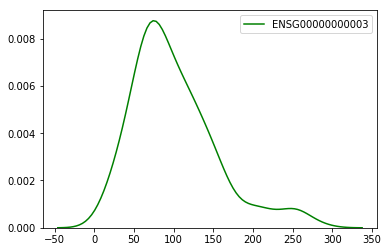``````
``````

# Differential Expression

``````import matplotlib.pyplot as plt
import seaborn as sns
import pandas
from scipy.stats import ttest_ind
import gripql
``````
``````conn = gripql.Connection("https://bmeg.io/api", credential_file="bmeg_credentials.json")
G = conn.graph("rc5")
``````

Look at the expression in the TCGA LUAD project

``````PROJECT="Project:TCGA-LUAD"
``````

Starting from the TCGA-LUAD project, follow the edges from `Project` -> `Case` -> `Sample` -> `Aliquot` -> `GeneExpression`. At the `Sample` node, we select from `Solid Tissue Normal` so we only pull the normals during this query.

Once on the gene expression node, extract the `values`, which hold the gene expression values in a Map[String,Float] format with Ensembl Gene ids as the keys, and the Gene expression TPMs as the values. We then load that into a Pandas data frame, and transpose so that the rows are the sample ids.

``````c = G.query().V(PROJECT).out("cases").out("samples").as_("sample")
c = c.has(gripql.eq("gdc_attributes.sample_type", "Solid Tissue Normal"))
c = c.out("aliquots").out("gene_expressions").as_("exp")
c = c.render( ["\$sample._data.gdc_attributes.submitter_id", "\$exp._data.values"])
data = {}
for row in c.execute(stream=True):
data[row] = row
normalDF = pandas.DataFrame(data).transpose()
``````
``````[INFO]  2020-01-14 14:07:38,218 59 results received in 12 seconds
``````

Do the Project to Gene Expression traversal again, but this time only select the tumor samples.

``````c = G.query().V(PROJECT).out("cases").out("samples").as_("sample")
c = c.has(gripql.eq("gdc_attributes.sample_type", "Primary Tumor"))
c = c.out("aliquots").out("gene_expressions").as_("exp")
c = c.render( ["\$sample._data.gdc_attributes.submitter_id", "\$exp._data.values"])
data = {}
for row in c.execute(stream=True):
data[row] = row
tumorDF = pandas.DataFrame(data).transpose()
``````
``````[INFO]  2020-01-14 14:09:27,596 539 results received in 107 seconds
``````

For each gene, run t-test to determine the genes with the most differential expression between the tumor and normal sets.

``````stats = {}
for gene in tumorDF:
s = ttest_ind(tumorDF[gene], normalDF[gene])
stats[gene] = { 'statistic': s.statistic, 'pvalue' : s.pvalue }
statsDF = pandas.DataFrame(stats).transpose()
``````

Display the results

``````statsDF[ statsDF['pvalue'] < 0.0001 ].sort_values('statistic').head()
``````
statistic pvalue
ENSG00000168484 -53.356952 6.389050e-227
ENSG00000135604 -48.643308 9.675744e-208
ENSG00000204305 -46.262534 1.283753e-197
ENSG00000114854 -44.158458 2.022993e-188
ENSG00000022267 -40.822561 2.395204e-173

Using the top gene from the T-Test experiment (ENSG00000168484), plot the expression of the gene across the tumor and normal samples

``````sns.kdeplot(tumorDF['ENSG00000168484'], color="r")
sns.kdeplot(normalDF['ENSG00000168484'], color="g")
``````
``````<matplotlib.axes._subplots.AxesSubplot at 0x11677b8d0>
``````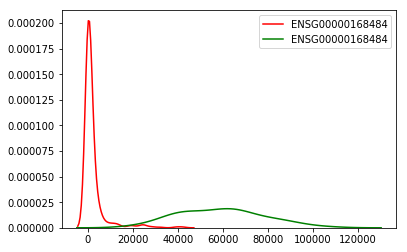Do a quick search of `ENSG00000168484` to identify the Gene Ontology terms that it is linked to

``````for row in G.query().V("ENSG00000168484").out("gene_ontology_terms"):
print(row.gid, row.data.definition)
``````
``````[INFO]  2020-01-14 14:10:14,516 10 results received in 0 seconds

GeneOntologyTerm:GO:0005515 Interacting selectively and non-covalently with any protein or protein complex (a complex of two or more proteins that may include other nonprotein molecules).
GeneOntologyTerm:GO:0005576 The space external to the outermost structure of a cell. For cells without external protective or external encapsulating structures this refers to space outside of the plasma membrane. This term covers the host cell environment outside an intracellular parasite.
GeneOntologyTerm:GO:0005615 That part of a multicellular organism outside the cells proper, usually taken to be outside the plasma membranes, and occupied by fluid.
GeneOntologyTerm:GO:0005789 The lipid bilayer surrounding the endoplasmic reticulum.
GeneOntologyTerm:GO:0007585 The process of gaseous exchange between an organism and its environment. In plants, microorganisms, and many small animals, air or water makes direct contact with the organism's cells or tissue fluids, and the processes of diffusion supply the organism with dioxygen (O2) and remove carbon dioxide (CO2). In larger animals the efficiency of gaseous exchange is improved by specialized respiratory organs, such as lungs and gills, which are ventilated by breathing mechanisms.
GeneOntologyTerm:GO:0042599 A membrane-bounded organelle, specialized for the storage and secretion of various substances (surfactant phospholipids, glycoproteins and acid phosphates) which are arranged in the form of tightly packed, concentric, membrane sheets or lamellae. Has some similar properties to, but is distinct from, a lysosome.
GeneOntologyTerm:GO:0042802 Interacting selectively and non-covalently with an identical protein or proteins.
GeneOntologyTerm:GO:0044267 The chemical reactions and pathways involving a specific protein, rather than of proteins in general, occurring at the level of an individual cell. Includes cellular protein modification.
GeneOntologyTerm:GO:0045334 A clathrin-coated, membrane-bounded intracellular vesicle formed by invagination of the plasma membrane around an extracellular substance.
GeneOntologyTerm:GO:0097486 The volume enclosed by the outermost membrane of a multivesicular body.
``````
``````
``````

# CNA Histogram

``````import matplotlib.pyplot as plt
import numpy as np
import gripql
conn = gripql.Connection("https://bmeg.io/api", credential_file="bmeg_credentials.json")
G = conn.graph("rc5")
``````

Get Ensembl Gene ids for genes of interest

``````GENES = ["PTEN", "TP53", "RB1"]
gene_ids = {}
for g in GENES:
for i in G.query().V().hasLabel("Gene").has(gripql.eq("symbol", g)):
gene_ids[g] = i.gid
``````
``````[INFO]  2020-01-14 13:44:13,074 1 results received in 0 seconds
[INFO]  2020-01-14 13:44:13,241 1 results received in 0 seconds
[INFO]  2020-01-14 13:44:13,426 1 results received in 0 seconds
``````
``````gene_ids
``````
``````{'PTEN': 'ENSG00000171862',
'TP53': 'ENSG00000141510',
'RB1': 'ENSG00000139687'}
``````

For each gene of interest, obtain the copy number alteration values and aggregate them by gene.

``````q = G.query().V("Project:TCGA-PRAD").out("cases").out("samples").out("aliquots")
q = q.has(gripql.eq("\$.gdc_attributes.sample_type", 'Primary Tumor')).out("copy_number_alterations")
q = q.aggregate(
list( gripql.term( g, "values.%s" % (g), 5) for g in gene_ids.values() )
)

res = list(q)
for r in res:
for b in res[r]['buckets']:
print("%s\t%s:%s" % (r, b['key'], b['value']))
``````
``````[INFO]  2020-01-14 13:44:23,255 1 results received in 3 seconds

ENSG00000139687 0:269
ENSG00000139687 -1:139
ENSG00000139687 -2:81
ENSG00000139687 1:3
ENSG00000141510 0:329
ENSG00000141510 -1:126
ENSG00000141510 -2:37
ENSG00000171862 0:327
ENSG00000171862 -2:95
ENSG00000171862 -1:64
ENSG00000171862 1:5
ENSG00000171862 2:1
``````

Create a barchart showing the counts of copy number altered samples in the cohort.

``````val = []
count = []
for i in sorted(res['ENSG00000139687']['buckets'], key=lambda x:int(x["key"])):
val.append(int(i["key"]))
count.append(i["value"])
plt.bar(val, count, width=0.35)
``````
``````<BarContainer object of 4 artists>
``````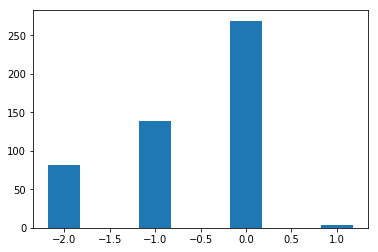``````
``````

# Drug Response

``````import pandas
import gripql
import itertools
import scipy.stats as stats

conn = gripql.Connection("https://bmeg.io/api", credential_file="bmeg_credentials.json")
G = conn.graph("rc5")
``````

Find all of the samples in the CTRP Breast Cancer experiment

``````q = G.query().V("Program:CTRP").out("projects").out("cases").\
has(gripql.eq("cellline_attributes.Primary Disease", "Breast Cancer")).distinct()
all_cases = []
for row in q:
all_cases.append(row.gid)
``````
``````[INFO]  2020-01-14 13:36:52,216 40 results received in 0 seconds
``````

For the genes of interest, get Ensembl gene ids, from the HUGO symbols

``````GENES = ["PTEN", "TP53"]
``````
``````gene_ids = {}
for i in G.query().V().hasLabel("Gene").has(gripql.within("symbol", GENES)):
gene_ids[i.data.symbol] = i.gid
``````
``````[INFO]  2020-01-14 13:36:56,003 2 results received in 0 seconds
``````

The CTRP doesn’t have direct mutation calling, but rather they used the same cell lines as the CCLE, and we can use the mutation calls from that project. So use the `same_as` edge to identify the cases from CCLE that are the same as the ones in CTRP. Then use the mutations from those samples.

``````gene_ids
``````
``````{'TP53': 'ENSG00000141510', 'PTEN': 'ENSG00000171862'}
``````

For each of the genes, find the set of samples that have a mutation in that gene

``````mut_cases = {}
norm_cases = {}

q = G.query().V(all_cases).as_("ctrp").out("same_as").has(gripql.eq("project_id", "Project:CCLE"))
q = q.out("samples").out("aliquots").out("somatic_callsets")
q = q.outE("alleles").has(gripql.within("ensembl_gene", list(gene_ids.values())))
q = q.render({"case" : "\$ctrp._gid", "gene" : "\$._data.ensembl_gene"})

for res in q:
mut_cases[res.gene] = mut_cases.get(res.gene, set()) | set([res.case])

#get CCLE samples without mutation
for i in gene_ids.values():
norm_cases[i] = list(set(all_cases).difference(mut_cases[i]))

print( "%s Positive Set: %d" % (i, len(mut_cases[i])) )
print( "%s Negative Set: %d" % (i, len(norm_cases[i])) )

``````
``````[INFO]  2020-01-14 13:39:36,629 43 results received in 0 seconds

ENSG00000141510 Positive Set: 29
ENSG00000141510 Negative Set: 11
ENSG00000171862 Positive Set: 9
ENSG00000171862 Negative Set: 31
``````
``````pos_response = {}
for g in gene_ids.values():
pos_response[g] = {}
q = G.query().V(list(mut_cases[g])).as_("a").out("samples").out("aliquots")
q = q.out("drug_response").as_("a").out("compounds").as_("b")
q = q.select(["a", "b"])
for row in q:
v = row['a']['data']['aac']
compound = row['b']['gid']
if compound not in pos_response[g]:
pos_response[g][compound] = [ v ]
else:
pos_response[g][compound].append(v)

``````
``````[INFO]  2020-01-14 13:39:55,140 12,224 results received in 3 seconds
[INFO]  2020-01-14 13:39:56,594 3,999 results received in 1 seconds
``````
``````neg_response = {}
for g in gene_ids.values():
neg_response[g] = {}
q = G.query().V(list(norm_cases[g])).as_("a").out("samples").out("aliquots")
q = q.out("drug_response").as_("a").out("compounds").as_("b")
q = q.select(["a", "b"])
for row in q:
v = row['a']['data']['aac']
compound = row['b']['gid']
if compound not in neg_response[g]:
neg_response[g][compound] = [ v ]
else:
neg_response[g][compound].append(v)

``````
``````[INFO]  2020-01-14 13:39:59,162 5,025 results received in 1 seconds
[INFO]  2020-01-14 13:40:03,457 13,250 results received in 4 seconds
``````
``````drugs = set(itertools.chain.from_iterable( i.keys() for i in pos_response.values() ))
out = []
for drug in drugs:
for g in gene_ids.values():
if drug in pos_response[g] and drug in neg_response[g]:
row = {"drug" : drug, "mutation" : g}
mut_values = pos_response[g][drug]
norm_values = neg_response[g][drug]
if len(mut_values) > 5 and len(norm_values) > 5:
s = stats.ttest_ind(mut_values, norm_values, equal_var=False)
row["t-statistic"] = s.statistic
row["t-pvalue"] = s.pvalue
s = stats.f_oneway(mut_values, norm_values)
row["a-statistic"] = s.statistic
row["a-pvalue"] = s.pvalue
out.append(row)
``````
``````pandas.DataFrame(out, columns=["drug", "mutation", "t-statistic", "t-pvalue", "a-statistic", "a-pvalue"]).sort_values("a-pvalue").head(10)
``````
drug mutation t-statistic t-pvalue a-statistic a-pvalue
277 Compound:CID216345 ENSG00000141510 -4.425335 0.000366 23.811104 0.000019
593 Compound:CID16736978 ENSG00000141510 -3.177435 0.006779 12.249536 0.001356
214 Compound:CID9967941 ENSG00000141510 -3.114875 0.006419 11.046079 0.002136
31 Compound:CID9549297 ENSG00000141510 -2.573574 0.021992 10.077463 0.003071
480 Compound:CID56949517 ENSG00000171862 2.235155 0.069114 10.480699 0.003638
808 Compound:CID5472285 ENSG00000141510 -2.711139 0.015176 9.369077 0.004037
566 Compound:NO_ONTOLOGY:CID9809715 ENSG00000141510 -2.492241 0.026354 8.810857 0.005631
237 Compound:CID451668 ENSG00000171862 2.030714 0.051533 7.157550 0.008627
95 Compound:CID54686904 ENSG00000171862 2.241852 0.067998 8.668157 0.009525
656 Compound:CID11609586 ENSG00000171862 1.948182 0.066221 6.818910 0.011089

# Kaplan Meier

Install the lifelines library

``````!pip install lifelines
``````
``````Collecting lifelines
[K     |████████████████████████████████| 409kB 4.1MB/s eta 0:00:01
Requirement already satisfied: scipy>=1.0 in /usr/local/lib/python3.7/site-packages (from lifelines) (1.3.1)
Collecting matplotlib>=3.0
Using cached https://files.pythonhosted.org/packages/a0/76/68bc3374ffa2d8d3dfd440fe94158fa8aa2628670fa38bdaf186c9af0d94/matplotlib-3.1.2-cp37-cp37m-macosx_10_9_x86_64.whl
Requirement already satisfied: numpy>=1.14.0 in /usr/local/lib/python3.7/site-packages (from lifelines) (1.17.3)
Requirement already satisfied: pandas>=0.23.0 in /usr/local/lib/python3.7/site-packages (from lifelines) (0.25.1)
Requirement already satisfied: pyparsing!=2.0.4,!=2.1.2,!=2.1.6,>=2.0.1 in /usr/local/lib/python3.7/site-packages (from matplotlib>=3.0->lifelines) (2.2.0)
Requirement already satisfied: python-dateutil>=2.1 in /usr/local/lib/python3.7/site-packages (from matplotlib>=3.0->lifelines) (2.7.3)
Requirement already satisfied: cycler>=0.10 in /usr/local/lib/python3.7/site-packages (from matplotlib>=3.0->lifelines) (0.10.0)
Requirement already satisfied: kiwisolver>=1.0.1 in /usr/local/lib/python3.7/site-packages (from matplotlib>=3.0->lifelines) (1.0.1)
Requirement already satisfied: pytz>=2017.2 in /usr/local/lib/python3.7/site-packages (from pandas>=0.23.0->lifelines) (2018.5)
Requirement already satisfied: six>=1.5 in /usr/local/lib/python3.7/site-packages (from python-dateutil>=2.1->matplotlib>=3.0->lifelines) (1.12.0)
Requirement already satisfied: setuptools in /usr/local/lib/python3.7/site-packages (from kiwisolver>=1.0.1->matplotlib>=3.0->lifelines) (42.0.2)
Building wheels for collected packages: autograd
Building wheel for autograd (setup.py) ... [?25ldone
Stored in directory: /Users/strucka/Library/Caches/pip/wheels/42/62/66/1121afe23ff96af4e452e0d15e68761e3f605952ee075ca99f
Found existing installation: matplotlib 2.2.2
Uninstalling matplotlib-2.2.2:
Successfully uninstalled matplotlib-2.2.2
``````
``````from lifelines import KaplanMeierFitter
import matplotlib.pyplot as plt

import pandas
import gripql

conn = gripql.Connection("https://bmeg.io/api", credential_file="bmeg_credentials.json")
G = conn.graph("rc5")
``````

Look at the TCGA-BRCA cohort, and find all of the cases where there is a recorded `days_to_death`

``````q = G.query().V("Project:TCGA-BRCA").out("cases")

data = {}
for i in q:
if i.data.gdc_attributes.demographic is not None and i.data.gdc_attributes.demographic.vital_status == "Dead":
if 'days_to_death' in i.data.gdc_attributes.demographic:
data[ i.gid ] = i.data.gdc_attributes.demographic.days_to_death
survival = pandas.Series(data)
``````
``````[INFO]  2020-01-14 14:18:49,810 1,098 results received in 0 seconds
``````

Gene ensembl gene id for `TP53`

``````gene = G.query().V().hasLabel("Gene").has(gripql.eq("symbol", "TP53")).execute().data.gene_id
print(gene)
``````
``````[INFO]  2020-01-14 14:19:08,643 1 results received in 0 seconds

ENSG00000141510
``````

Starting from the cases with attached survival information, find all of the cases that have a mutation in the gene on interest

``````q = G.query().V(list(survival.keys())).as_("case").out("samples").out("aliquots").out("somatic_callsets").out("alleles")
q = q.has(gripql.eq("ensembl_gene", gene))
q = q.select("case").distinct("\$._gid").render("\$._gid")
mut_cases = list(q)
``````
``````[INFO]  2020-01-14 14:19:47,044 52 results received in 2 seconds
``````

Plot a Kaplan Meirer curve to demonstrate the different in survival of the somatic mutation group and those with no somatic mutation.

``````kmf = KaplanMeierFitter()

ax = plt.subplot(111)
kmf.fit(survival[mut_cases], label="%s Mutations" % (gene))
ax = kmf.plot(ax=ax)
kmf.fit(survival[ survival.index.difference(mut_cases) ], label="No Mutation")
kmf.plot(ax=ax)
``````
``````<matplotlib.axes._subplots.AxesSubplot at 0x1205a8d10>
``````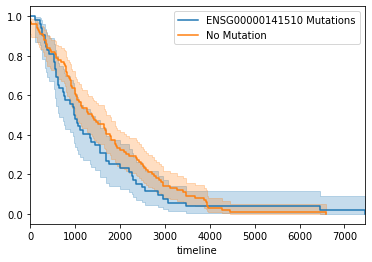``````
``````